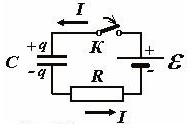homechevron_rightStudychevron_rightPhysics

# Capacitor in Direct Current Circuit

These online calculators computes various parameters for charging and discharging the capacitor with the resistor

### This page exists due to the efforts of the following people:

#### Timur

These online calculators computes various parameters for charging and discharging the capacitor with the resistor. Formulae used for calculations are below the calculators.#### Charging the Capacitor with a Resistor

Digits after the decimal point: 2
Time Constant, milliseconds

5 Time Constants (99.2% charged), milliseconds

Initial Current, Amperes

Maximum Power Dissipation, Watts

Capacitor Voltage, Volts

Capacitor Charge, microCoulombs

Capacitor Energy, milliJoules

Power Supply Work, milliJoules#### Discharging the Capacitor with the Resistor

Digits after the decimal point: 2
Initial Capacitor Energy, milliJoules

Initial Capacitor Charge, microCoulombs

Time Constant, milliseconds

Initial Current, Amperes

Maximum Power Dissipation, Watts

Final Capacitor Charge, microCoulombs

Final Capacitor Energy, milliJoules

Final Capacitor Voltage, Volts

Below is the picture of electrical circuit for charging the capacitor with the power supply unit.After switch K is closed, direct current starts charging the capacitor.
According to Ohms law, the sum of capacitor and resistor voltages is equal to power supply voltage.

The capacitor charge and current depend on time. At the initial moment, there is no charge at the capacitor, thus, current is maximum, as well as power dissipation on the resistor.

During charging, capacitor voltage changing according to the following equation

where tau

is called Time Constant. Since charging is infinite process, usually, a capacitor is considered to be fully charged after 5 time constants. After 5 time constants, the capacitor will be charged to 99.2% of the supply voltage.
Capacitor Charge

Capacitor Energy

Work of Power Supply

URL copied to clipboardPLANETCALC, Capacitor in Direct Current Circuit